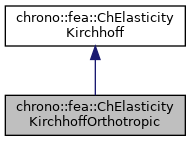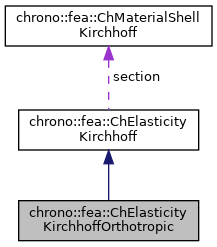chrono::fea::ChElasticityKirchhoffOrthotropic Class Reference

## Description

Orthotropic elasticity for thin shells (Kirchoff-Love shell theory, without shear effects) to be used in a ChMaterialShellKirchhoff.

This class implements material properties for a layer of a Kirchhoff thin shell, for the case of orthotropic linear elastic material. This is useful for laminated shells. One direction can be made softer than the other. Note that the angle and the thickness are defined when adding a material with this elasticity to a shell finite element as a layer.

#include <ChMaterialShellKirchhoff.h>

Inheritance diagram for chrono::fea::ChElasticityKirchhoffOrthotropic:[legend]
Collaboration diagram for chrono::fea::ChElasticityKirchhoffOrthotropic:[legend]

## Public Member Functions

ChElasticityKirchhoffOrthotropic (double m_E_x, double m_E_y, double m_nu_xy, double m_G_xy)
Construct an orthotropic material. More...

ChElasticityKirchhoffOrthotropic (double m_E, double m_nu)
Construct an orthotropic material as sub case isotropic. More...

double Get_E_x () const
Return the elasticity moduli, on x.

double Get_E_y () const
Return the elasticity moduli, on y.

double Get_nu_xy () const
Return the Poisson ratio, for xy.

double Get_nu_yx () const
Return the Poisson ratio, for yx (follows xy as it must be nu_yx*E_x = nu_xy*E_y)

double Get_G_xy () const
Return the shear mod, in plane.

virtual void ComputeStress (ChVector<> &n, ChVector<> &m, const ChVector<> &eps, const ChVector<> &kur, const double z_inf, const double z_sup, const double angle) override
The FE code will evaluate this function to compute per-unit-length forces/torques given the strains/curvatures. More...

virtual void ComputeStiffnessMatrix (ChMatrixRef mC, const ChVector<> &eps, const ChVector<> &kur, const double z_inf, const double z_sup, const double angle) override
Compute 6x6 stiffness matrix [Km] , that is [ds/de], the tangent of the constitutive relation per-unit-length forces/torques vs generalized strains. More...

## Additional Inherited MembersPublic Attributes inherited from chrono::fea::ChElasticityKirchhoff
ChMaterialShellKirchhoffsection

## ◆ ChElasticityKirchhoffOrthotropic() [1/2]

 chrono::fea::ChElasticityKirchhoffOrthotropic::ChElasticityKirchhoffOrthotropic ( double m_E_x, double m_E_y, double m_nu_xy, double m_G_xy )

Construct an orthotropic material.

Parameters
 m_E_x Young's modulus on x m_E_y Young's modulus on y m_nu_xy Poisson ratio xy (for yx it holds: nu_yx*E_x = nu_xy*E_y) m_G_xy Shear modulus, in plane

## ◆ ChElasticityKirchhoffOrthotropic() [2/2]

 chrono::fea::ChElasticityKirchhoffOrthotropic::ChElasticityKirchhoffOrthotropic ( double m_E, double m_nu )

Construct an orthotropic material as sub case isotropic.

Parameters
 m_E Young's modulus on x m_nu Poisson ratio

## ◆ ComputeStiffnessMatrix()

 void chrono::fea::ChElasticityKirchhoffOrthotropic::ComputeStiffnessMatrix ( ChMatrixRef mC, const ChVector<> & eps, const ChVector<> & kur, const double z_inf, const double z_sup, const double angle )
overridevirtual

Compute 6x6 stiffness matrix [Km] , that is [ds/de], the tangent of the constitutive relation per-unit-length forces/torques vs generalized strains.

Parameters
 mC tangent matrix eps strains e_11, e_22, e_12 kur curvature k_11, k_22, k_12 z_inf layer lower z value (along thickness coord) z_sup layer upper z value (along thickness coord) angle layer angle respect to x (if needed)

Reimplemented from chrono::fea::ChElasticityKirchhoff.

## ◆ ComputeStress()

 void chrono::fea::ChElasticityKirchhoffOrthotropic::ComputeStress ( ChVector<> & n, ChVector<> & m, const ChVector<> & eps, const ChVector<> & kur, const double z_inf, const double z_sup, const double angle )
overridevirtual

The FE code will evaluate this function to compute per-unit-length forces/torques given the strains/curvatures.

Parameters
 n forces n_11, n_22, n_12 (per unit length) m torques m_11, m_22, m_12 (per unit length) eps strains e_11, e_22, e_12 kur curvature k_11, k_22, k_12 z_inf layer lower z value (along thickness coord) z_sup layer upper z value (along thickness coord) angle layer angle respect to x (if needed) -not used in this, isotropic

Implements chrono::fea::ChElasticityKirchhoff.

The documentation for this class was generated from the following files:
• /builds/uwsbel/chrono/src/chrono/fea/ChMaterialShellKirchhoff.h
• /builds/uwsbel/chrono/src/chrono/fea/ChMaterialShellKirchhoff.cpp